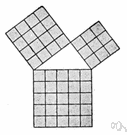# theorem

(redirected from Corrolary)
Also found in: Thesaurus, Medical, Legal, Encyclopedia.

## the·o·rem

(thē′ər-əm, thîr′əm)
n.
1. An idea that has been demonstrated as true or is assumed to be so demonstrable.
2. Mathematics A proposition that has been or is to be proved on the basis of explicit assumptions.

[Late Latin theōrēma, from Greek, from theōrein, to look at, from theōros, spectator; see theory.]

## theorem

(ˈθɪərəm)
n
(Logic) maths logic a statement or formula that can be deduced from the axioms of a formal system by means of its rules of inference
[C16: from Late Latin theōrēma, from Greek: something to be viewed, from theōrein to view]
theorematic, theoremic, theorematical adj

## the•o•rem

(ˈθi ər əm, ˈθɪər əm)

n.
1. Math. a theoretical proposition, statement, or formula embodying something to be proved from other propositions or formulas.
2. a rule or law, esp. one expressed by an equation or formula.
3. Logic. a proposition that can be deduced from the premises or assumptions of a system.
4. an idea, method, or statement generally accepted as true or worthwhile without proof.
[1545–55; < Late Latin theōrēma < Greek theṓrēma spectacle, object of contemplation, theorem =theōrē-, variant s. of theōreîn to observe, derivative of theōrós person sent to consult an oracle, spectator + -ma resultative n. suffix]
the`o•re•mat′ic (-əˈmæt ɪk) adj.

## the·o·rem

(thē′ər-əm, thîr′əm)
A mathematical statement whose truth can be proved on the basis of a given set of axioms or assumptions.
ThesaurusAntonymsRelated WordsSynonymsLegend:
 Noun 1theorem - a proposition deducible from basic postulatesbinomial theorem - a theorem giving the expansion of a binomial raised to a given powerproposition - (logic) a statement that affirms or denies something and is either true or false 2 theorem - an idea accepted as a demonstrable truthidea, thought - the content of cognition; the main thing you are thinking about; "it was not a good idea"; "the thought never entered my mind"Bayes' theorem - (statistics) a theorem describing how the conditional probability of a set of possible causes for a given observed event can be computed from knowledge of the probability of each cause and the conditional probability of the outcome of each cause

## theorem

noun He postulated a theorem and proved it.

## theorem

noun
A broad and basic rule or truth:
Translations
نَظَرِيَّه
teorém
læresætning
elméleti tétel
setning
teorema
teorēma
teoréma
davateorem

## theorem

[ˈθɪərəm] N (Math) →

[ˈθiːərəm] n

## theorem

nSatz m (also Math), → Theorem nt (geh, spec)

## theorem

[ˈθɪərəm] n (Math) →

## theorem

(ˈθiərəm) noun
especially in mathematics, something that has been or must be proved to be true by careful reasoning. a geometrical theorem.
References in periodicals archive ?
158) The concurrence, meanwhile, invoked a corrolary to the democracy canon to resolve the question of whether a rented-out home could constitute a permanent abode in favor of ballot access, its establishment in prior Illinois case law making its invocation more legitimate.
And as a corrolary, why do some executives succeed in situations where others fail?
This notion, wonderfully reminiscent of Ranke, is made less convincing, however, by the corrolary argument that the sense of community is created by religion demonizing the "Other.

Site: Follow: Share:
Open / Close